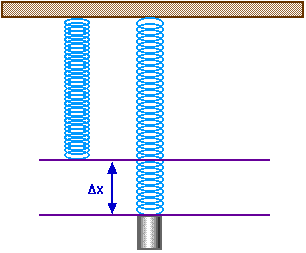# Counting swings of a spring!?

Determining the gravity acceleration via damping spring is one of the fundamental physics experiments. Because students can not start stopwatch on time and also detecting the exact moment of transition of the the spring at a given point is difficult, often quite unexpected results of this trial will be appeared.
We decided to find a way to count the number of (e.g 10) swings, with the help of an eye-sensor.
That is:

• Connecting to an electronic stopwatch
• Connecting to an optical sensor
• Programming to calculate the 10 swings

Such a thing is possible with arduino? Is it done before? sth similar will be a help!

Let the spring hang from a load cell and the Arduino measuring the weight 10 to 100 times a second.

Can something be attached to the spring ? or will the weight have influence ? Something can be connected that bounces the signal of an ultrasonic or IR distance sensor. Or an accelerometer directly connected to the spring.

I would use a IR distance sensor under the spring.
Like this

Or you could use a pendulum like my project here:-

for the stopwatch try - Arduino Playground - StopWatchClass -

Peter_n:
Let the spring hang from a load cell and the Arduino measuring the weight 10 to 100 times a second.

Which kind of load cells? there's different types: Load cell - Wikipedia

Can something be attached to the spring ?

A hanged spring with weights. Students increase the weights and measure the time of 10 swings per weight.or will the weight have influence ?

?

Or an accelerometer directly connected to the spring.

:~
Students should compute the acceleration by the date.

Grumpy_Mike:
I would use a IR distance sensor under the spring.

Under the spring? (does it work by maximum distance?), can it count 10 swings?

Grumpy_Mike:
Or you could use a pendulum like my project here:-
Harmonograph on Vimeo

What's the kind of its sensors?

Under the spring?

Yes.

does it work by maximum distance?

It measures distance continuously, you want to measure the minimum distance, that is what your code should do.

can it count 10 swings?

that is what your code should do.

Students should compute the acceleration by the date.

By looking at the data you can tell when the maximum and minimum points are. If you know the rate of the data measurements you can determine the time.

Use an IR distance sensor. I think that is the easiest solution and it will work.

There might be many other ways (load cell, magnet + hall, ultrasonic distance, accelerometer, visual with webcam + software, IR gate), but they are not better.

Peter_n:
Use an IR distance sensor. I think that is the easiest solution and it will work.

Is this what you mean:

Is this what you mean:

No no no no no no no no no!!!!!!

Use a voice recognition library and count how many times the string "boing" occurs in the output.

A 'real' IR proximity sensor.
For example these:

You have to find one that has a range that you need and works with 5V.

So all you need is an Arduino Uno and such a sensor (and a 7...7.5...12V power supply). Connect the Arduino Uno to a computer, make the code for it, and maybe you have to attach a piece of white paper to the bottom of the weights.

Have a look at the datasheet, so you know what the output graph is according to the distance.

The Power Supply can be a small switching power supply. It might be needed for more accurate data. The Arduino uses the 5V by default for reference to measure an analog voltage. When the Arduino Uno is powered via the usb cable, the 5V could be 4.6V, and that makes the data inaccurate. You can avoid that by powering the Arduino Uno with a power supply to the barrel jack.